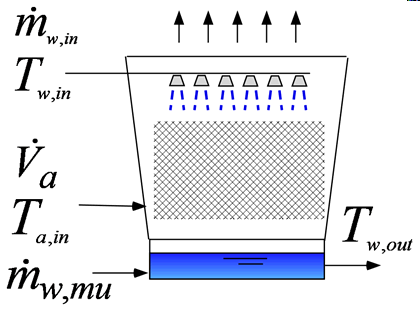Contents - index

CoolingTower1_CLThis procedure determines the performance of a counterflow cooling tower using the analogy method described in Mitchell and Braun, Heating, Ventilation, and Air Conditioning in Buildings, Chapter 14, 2013.  An enthalpy effectiveness is determined as a function of the heat transfer coefficient between the air and the water.  Fan power is not calculated and its effect on the tower capacity is not included.  Drift  or water spray carryover is neglected.  Since the makeup water flow rate is small relative to the flow rate of water entering the tower, the effect of makeup water temperature is neglected.

Inputs:

P_atm:  atmospheric pressure (Pa, kPa, bar, MPa, psia, atm)

V_dot_a:  volumetric flow rate of entering air (m^3/s, cfm)

T_a_in:  temperature of entering air (C, K, F, R)

m_dot_w:  mass flow rate of entering warm water (kg/s, lbm/hr)

T_w_in:  temperature of entering water (C, K, F, R)

UA:  overall heat transfer coefficient - area product (W/K, Btu/hr-F)

Outputs:

T_w_out temperature of water returning from the cooling tower (C, K, F, R)

m_w_mu rate at which makeup water must be supplied to the cooling tower (kg/s, lbm/hr)

Capacity:   product of the mass flow rate of water and its enthalpy change (W, Btu/hr)

Range:  difference between the inlet and outlet water temperatures (C, K, F, R)

Approach:  difference between the outlet water and the wetbulb temperatures (C, K, F, R)

Example:

\$UnitSystem Eng psia F mass

T_a_in=85 [F] "inlet air temperature"

RH_a_in = 0.50 "inlet air relative humidity"

P_atm = 14.7 [psia] "inlet air pressure"

V_dot_a = 6000 [ft^3/min] "volumetric flow rate of air into tower"

RH_a_out=1 "assumed relative humidity at outlet of tower"

T_w_in = 100 [F] "inlet water temperature"

m_dot_w_in=30000 [lb_m/hr] "inlet water flow rate"

h_v=6 [Btu/h-ft^2-F] "heat transfer coefficient per units volume"

Vol=900 [ft^3] "volume of the cooling tower"

A=3 [ft^2/ft^3] "surface area per unit volume of the fill material"

UA=h_v*Vol*A "overall heat transfer coefficient - area product"

Call coolingtower1_cl(P_atm, V_dot_a, T_a_in, RH_a_in, m_dot_w_in, T_w_in, UA: T_w_out, m_dot_mu, Capacity, Range, Approach)

{Solution:

Approach=8.767 [F]

Capacity=612544 [Btu/hr]

m_dot_mu=536.6 [lb_m/hr]

Range=20.45 [F]

T_w_out=79.55 [F]}